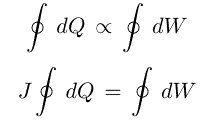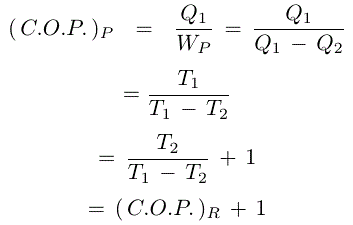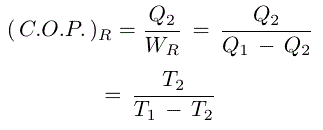# Types Of Thermodynamics laws And It’s Application

In this page, we discuss different types of laws of thermodynamics and their importance in practical field.

Following thermodynamics laws are important

1. Zeroth law of thermodynamics
2. First law of thermodynamics
3. Second law of thermodynamics
4. Third law of thermodynamics

## 1. Zeroth law of thermodynamics:-

Zeroth law of thermodynamics states that when two systems are each in thermal equilibrium with the third system, they are also in thermal equilibrium with each other.Above figure shows three systems A,B and C. If system A is in thermal equilibrium with the system C and system B is in thermal equilibrium with the system C then the system A is in thermal equilibrium with system B.

This law provides the basis of temperature measurement.

## 2. First law of thermodynamics:-

First law of thermodynamics concerns principle of conservation of energy. According to the conservation of energy, Energy can neither be created nor destroyed.During any process, It changes from one form to another but there is no change in the total energy of the system and its surroundings.

From the above statement, the first law of thermodynamics states that when a system undergoes a thermodynamic cycle then the net heat supplied to the system from its surroundings is proportional to the network done by the system on its surroundings.Where,∳dW = Network delivered to the surrounding during the cycle process.

Where,∳dQ = Net heat taken from the surrounding during the cycle process.

The constant J is called jule mechanical equivalent of heat.

### Limitations of first law of thermodynamics:-

In first law of thermodynamics,it is clearly stated that heat and work are converted one to another during any thermodynamic cycle of a closed system. But this statement does not satisfy all the situation. It does not specify the direction of flow of heat and work. Let,

I) Two bodies which are different individual temperature is like T and t and they are in contact each other but are separated from their surroundings and T >t ,then we know that heat flow from T to t till the temperature of both bodies are equal.The reversible process is not possible.It means the first law of thermodynamics does not mention whether the heat flows from a hot body to a cold body or a cold body to hot body.

II) Another practical experience,we see in automobile field.This law states that heat energy and mechanical work are mutually convertible.It means,heat energy and mechanical energy both are fully converted with each other.but practicalły it does not happen.When an automobile runs with a certain amount of speed and suddenly needs to stop,then full mechanical energy is converted into heat energy and the breaks get hot.In this case,first law of thermodynamics is fully satisfied.After a certain period breaks are getting cool and as per the law this breaks heat energy should be fully converted into mechanical energy to the automobile,it would cause the automobile to move on the road again.But practically it is impossible. A machine which does not satisfy the first law of thermodynamics is called Perpetual motion machine of the first kind or PMM-I.Actually,this type of machine is impossible.Because no machine produces energy without taking any other form of energy. From the above two reasons, it is clear that there are some lacking or limitation behind the first law of thermodynamics.The second law of thermodynamics full fill this limitation.

## 3. Second law of thermodynamics:-

The second law of thermodynamics can be described by the following two theories.

I) Kelvin-Planck Statement

II) Clausius Statement

### I) Kelvin-Planck Statement:-

According to the Kelvin-Planck's statement,no engine can be constructed in the world working with cyclic process.It means,no cycle process is possible whose result is the flow of heat from a single heat reservoir and the performance of an equivalent amount of work on a work reservoir.So,no heat engine can be covert his full heat energy into equivalent mechanical work.Actually a small part of the heat supplied to the engine is converted into mechanical work while large part of the heat is lost to its surroundings.This reason,Kelvin-Planck statement of the second law of thermodynamics,is sometimes known as law of degradation of energy. Basically,an engine which violates the second law of thermodynamics, is known as perpetual motion machine of the second kind or PMM-2 or 100% efficient machine.But practically construction of this machine is impossible.In the above fig.heat is supplied from the hot reservoir or source and part of heat is rejected to the cold reservoir or sink.In this case,heat energy from source Q1 at a temperature T1 is supplied to the engine and consequently part of heat energy Q2 is rejected to the sink with some temperature T2.So remaining heat (Q1-Q2) is converted into mechanical work.Therefore maximum thermal efficiency is the ratio of the maximum mechanical work obtained to the total heat supplied to the engine.Mathematically,

### II) Clausius Statement:-

No cyclic process is possible whose result is to transfer heat from a body at a lower temperature to a body at a higher temperature without the aid of an external agency.So heat energy can not be transfer from cold body to a hot body without acting any external source.In the above figure heat pump and refrigerator,both engines break Clausius statement theory.Because this type of supply heat from a cold body to hot body without taking any external help.This type of device is called perpetual motion machine of the second kind. Refrigerator and heat engine both are transferring heat from cold body to hot body in cyclic process but in different surroundings.Refrigerator operating in a cyclic process,maintain a cold body at a temperature lower than the temperature of the outside temperature.Heat pump operating in a cyclic process,maintain a hot body at temperature higher than the temperature of the outside temperature. From the above fig,mathematically maximum coefficient of performance for a refrigerator,Maximum coefficient of performance for a heat pump## 4. Third law of thermodynamics:-

Unattainability of absolute zero is based on the third law of thermodynamics which states that the entropy of a pure substance of absolute zero temperature is zero.This is also called Nernst Theorem.